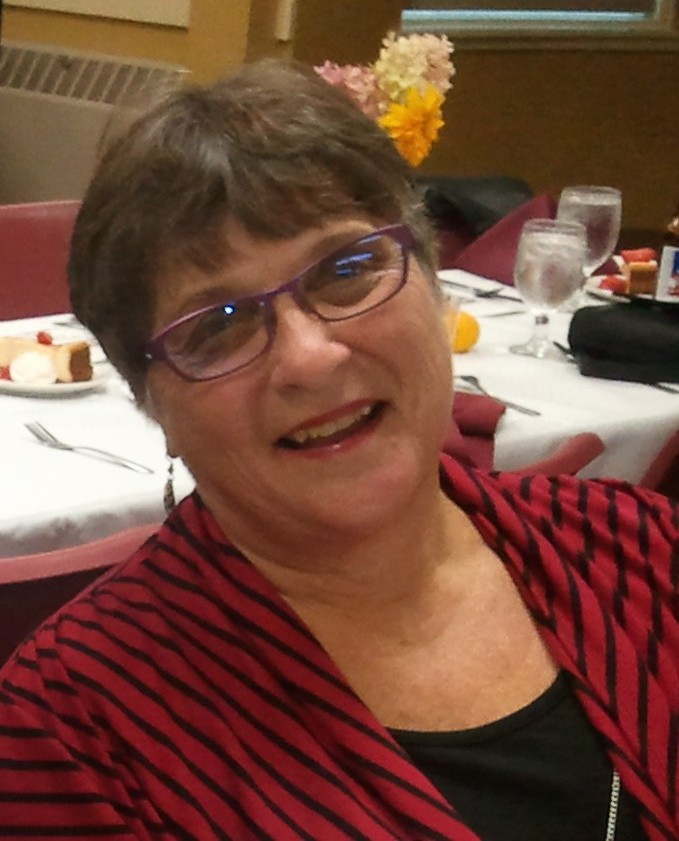## Utilizing Correct Mathematical Language

The Career and College Readiness Standards have shifted the way we teach mathematics to our students.  One change is the importance of using correct mathematical language for both our students and our teachers. In Mathematical Practice 1, students need to make sense of the mathematical language in order to problem solve. With Mathematical Practice 3, students need math language to discuss their ideas or critique their classmates’ ideas. With Mathematical Practice 6, students need to use the mathematical language in writing as well as in their heads.

Last week, the listserves were lit up with a new research article Supporting Clear and Concise Mathematical Language: Instead of That, Say This by researchers Elizabeth M. Hughes, Sarah R. Powell, and Elizabeth A. Stevens.1 These researchers feel that language plays an important role in learning mathematics. They suggest that teachers may not interpret mathematics as a second or third language for students when in fact, all students are mathematical language learners. That resonated with me as I think of all our ELL/ESOL teachers and their approach to learning vocabulary.  In their classes, vocabulary isn’t a list of words to memorize but rather a list of words to experience and understand through role play, etc.  The same is true in a math class. New vocabulary should be addressed as the learning experience happens through using manipulatives, in mathematical inquiries, or through discussion. What the article revealed was that we should be using the correct math terminology when the situation appears in the classroom. The authors gave many suggestions of when teachers might “say that, not this”. I will discuss a few of them by relating their ideas to an adult education class.

One suggestion was to stress the difference between number and digit when teaching place value. For example, 235 is a number, while the 2 is a digit in the hundreds place. This could be done by using base ten blocks where the student represents the number 235 using 2 hundred blocks, 3 ten rods and 5 unit blocks. They could see the visual representation of the number 235. They could also see the value of the digit 2 as 200, the digit 3 as 30, and the digit 5 as 5 ones. You could also represent the number 235 on a number line to show how close it is to 200 or 300. The point is that the words number and digit can have some conceptual understanding if utilizing manipulatives and number lines.

Other suggestions centered on fractions.  Too often we refer to the parts of a fraction as “the top number” and “the bottom number”. This suggests that the fraction is composed of two different numbers instead of digits that together are one number. This results in confusion for students. I can remember one of my students claiming that 3/4 and 4/5 were equal. When I asked her to explain why, she said that because 3 + 1 = 4 and 4 + 1 = 5, it was the same increase of 1 to arrive at 4/5, therefore the fractions were equal. Clearly the student was looking at each part separately and not thinking that 3/4 itself was a fractional number. The researchers suggest that we refer to the parts of the fraction by their correct names, numerator and denominator. I can remember hesitating to use these words with a low level math students for fear I would sound too technical. The point is again to introduce these terms more conceptually. Use fraction strips so students can develop understanding of what the numerator and denominator represent, and illustrate fraction as a number by locating it on a number line.

While both of these researchers based their work on elementary students, I find their suggestions very applicable to the adult education classroom as well. One detail they stressed was that most tests (such as the HiSET in adult ed), utilize more sophisticated math language. Students exposed to this language conceptually in a problem-based classroom develop a deeper conceptual understanding and perform better on standardized tests.

These are just two of many examples the researchers shared. To get the full report go to: http://bit.ly/2dUVkLH and request a copy.

###### 1 Hughes, E.M., Powell, S. R., & Stevens, E.A. (2016). Supporting Clear and Concise Mathematical Language: Instead of That, Say This. TEACHING Exceptional Children, Vol. 49(1), 7–17.

=============================================Pam Meader, a former high school math teacher, has taught math in adult education for over 25 years. She is a math consultant for the SABES PD Center for Mathematics and Adult Numeracy professional development initiative for Massachusetts. Most recently, she helped co-develop Adults Reaching Algebra Readiness (AR)2 with Donna Curry. She is a national trainer for LINCS and ANI (Adult Numeracy Instruction). Pam enjoys sharing techniques for teaching math conceptually from Basic Math through Algebra and has co-authored the Hands On Math series for Walch Publishing in Portland, Maine.